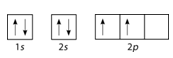# 3.9: Electron Configurations

$$\newcommand{\vecs}{\overset { \rightharpoonup} {\mathbf{#1}} }$$ $$\newcommand{\vecd}{\overset{-\!-\!\rightharpoonup}{\vphantom{a}\smash {#1}}}$$$$\newcommand{\id}{\mathrm{id}}$$ $$\newcommand{\Span}{\mathrm{span}}$$ $$\newcommand{\kernel}{\mathrm{null}\,}$$ $$\newcommand{\range}{\mathrm{range}\,}$$ $$\newcommand{\RealPart}{\mathrm{Re}}$$ $$\newcommand{\ImaginaryPart}{\mathrm{Im}}$$ $$\newcommand{\Argument}{\mathrm{Arg}}$$ $$\newcommand{\norm}{\| #1 \|}$$ $$\newcommand{\inner}{\langle #1, #2 \rangle}$$ $$\newcommand{\Span}{\mathrm{span}}$$ $$\newcommand{\id}{\mathrm{id}}$$ $$\newcommand{\Span}{\mathrm{span}}$$ $$\newcommand{\kernel}{\mathrm{null}\,}$$ $$\newcommand{\range}{\mathrm{range}\,}$$ $$\newcommand{\RealPart}{\mathrm{Re}}$$ $$\newcommand{\ImaginaryPart}{\mathrm{Im}}$$ $$\newcommand{\Argument}{\mathrm{Arg}}$$ $$\newcommand{\norm}{\| #1 \|}$$ $$\newcommand{\inner}{\langle #1, #2 \rangle}$$ $$\newcommand{\Span}{\mathrm{span}}$$$$\newcommand{\AA}{\unicode[.8,0]{x212B}}$$Figure $$\PageIndex{1}$$ (Credit: David R. Tribble (User:Loadmaster/Wikimedia Commons); Source: Commons wikimedia, Dir Command in Windows Command Prompt(opens in new window) [commons.wikimedia.org]; License: Public Domain)

### How big is a file?

If you keep your papers in manila folders, you can pick up a folder and see how much it weighs. If you want to know how many different papers (articles, bank records, or whatever else you keep in a folder), you have to take everything out and count. A computer directory, on the other hand, tells you exactly how much you have in each file. We can get the same information on atoms. If we use an orbital filling diagram, we have to count arrows. When we look at electron configuration data, we simply add up the numbers.

## Electron Configurations

Electron configuration notation eliminates the boxes and arrows of orbital filling diagrams. Each occupied sublevel designation is written followed by a superscript that is the number of electrons in that sublevel. For example, the hydrogen configuration is $$1s^1$$, while the helium configuration is $$1s^2$$. Multiple occupied sublevels are written one after another. The electron configuration of lithium is $$1s^2 2s^1$$. The sum of the superscripts in an electron configuration is equal to the number of electrons in that atom, which is in turn equal to its atomic number.

##### Example $$\PageIndex{1}$$

Draw the orbital filling diagram for carbon and write its electron configuration.

###### Known
• atomic number of carbon, Z=6

Use the order of fill diagram to draw an orbital filling diagram with a total of six electrons. Follow Hund's rule. Write the electron configuration.

###### Step 2: Construct diagram.Figure $$\PageIndex{2}$$: Orbital filling diagram for carbon. (Credit: Joy Sheng; Source: CK-12 Foundation; License: CC BY-NC 3.0(opens in new window))

Electron configuration $$1s^2 2s^2 2p^2$$

Following the $$2s$$ sublevel is the $$2p$$, and $$p$$ sublevels always consist of three orbitals. All three orbitals need to be drawn even if one or more is unoccupied. According to Hund's rule, the sixth electron enters the second of those $$p$$ orbitals, with the same spin as the fifth electron.

## Second Period Elements

Periods refer to the horizontal rows of the periodic table. The first period of the periodic table contains only the elements hydrogen and helium. This is because the first principal energy level consists of only the $$s$$ sublevel, and so only two electrons are required in order to fill the entire principal energy level. Each time a new principal energy level begins, as with the third element lithium, a new period is started on the periodic table. As one moves across the second period, electrons are successively added. With beryllium $$\left( Z=4 \right)$$, the $$2s$$ sublevel is complete and the $$2p$$ sublevel begins with boron $$\left( Z=5 \right)$$. Since there are three $$2p$$ orbitals and each orbital holds two electrons, the $$2p$$ sublevel is filled after six elements. Table $$\PageIndex{1}$$ shows the electron configurations of the elements in the second period.

Element Name Symbol Atomic Number Electron Configuration
Table $$\PageIndex{1}$$: Electron Configurations of Second-Period Elements
Lithium $$\ce{Li}$$ 3 $$1s^2 2s^1$$
Beryllium $$\ce{Be}$$ 4 $$1s^2 2s^2$$
Boron $$\ce{B}$$ 5 $$1s^2 2s^2 2p^1$$
Carbon $$\ce{C}$$ 6 $$1s^2 2s^2 2p^2$$
Nitrogen $$\ce{N}$$ 7 $$1s^2 2s^2 2p^3$$
Oxygen $$\ce{O}$$ 8 $$1s^2 2s^2 2p^4$$
Fluorine $$\ce{F}$$ 9 $$1s^2 2s^2 2p^5$$
Neon $$\ce{Ne}$$ 10 $$1s^2 2s^2 2p^6$$

## Summary

• Electron configuration notation simplifies the indication of where electrons are located in a specific atom.
• Superscripts are used to indicate the number of electrons in a given sublevel.

## Review

1. What does electron configuration notation eliminate?
2. How do we know how many electrons are in each sublevel?
3. An atom has the electron configuration of $$1s^2 2s^2 2p^5$$. How many electrons are in that atom?
4. Which element has the electron configuration of $$1s^2 2s^2 2p^6 3s^2$$?

3.9: Electron Configurations is shared under a CC BY-NC license and was authored, remixed, and/or curated by LibreTexts.